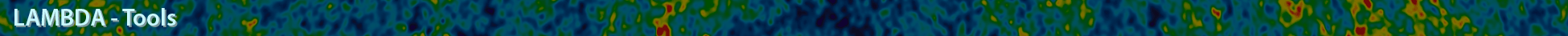# Low Mass and High Mass Halo Catalogs

```Cosmological parameters are based closely on the five year WMAP data release.

nsinit  = 0.96
hubble0 = 0.71
wde     = -1.0
sigma8  = 0.80
omegam  = 0.264
omegal  = 0.736 = 1-omegam
omegab  = 0.044
YHe     = 0.24
XH      = 0.76
Tcmb    = 2.726

The TPM N-body code was run with 1024^3 particles in a 1000 Mpc/h box. Halos were identified using a
friends-of-friends algorithm and those belonging to a light cone spanning an octant for redshifts 0 < z < 3 have
been saved.

TPM N-body simulation
L  = 1000 Mpc/h
Np = 1024^3
mp = 6.82E10 Msolar/h = 9.61E10 Msolar

FoF halo finder 1
bfof  = 0.2 times mean interparticle spacing
Nmin  = 293 particles
Mmin  = 2.00E13 Msolar/h = 2.82E13 Msolar
Nhalo = 1414339

FoF halo finder 2
bfof  = 0.2 times mean interparticle spacing
Nmin  = 100 particles
Nmax  = 292 particles
Mmin  = 6.82E12 Msolar/h = 9.61E12 Msolar
Mmax  = 1.99E13 Msolar/h = 2.80E13 Msolar
Nhalo = 6431049

Nbody halo catalogs are available in ascii and binary formats. For each halo, there are 20 numbers as described
below.

Here is a sample codelet to read in the binary data file

integer(4), parameter           :: nhalo = 1414339
real(4),    dimension(20,nhalo) :: halo

open(1,file='halo_nbody.binary',form='binary')
do i=1,nhalo
enddo
close(1)

Halo array
halo(    1,i) = redshift
halo(    2,i) = ra  in degrees
halo(    3,i) = dec in degrees
halo(  4:6,i) = comoving position of halo potential minimum in Mpc
halo(  7:9,i) = proper peculiar velocity in km/s
halo(   10,i) = Mfof in Msolar
halo(   11,i) = Mvir in Msolar
halo(   12,i) = Rvir in proper Mpc
halo(13:14,i) = M200,  R200
halo(15:16,i) = M500,  R500
halo(17:18,i) = M1500, R1500
halo(19:20,i) = M2500, R2500

In the halo_low_mass files, the halo properties (M1500,R1500,M2500,R2500) are set to zero because the resolution
is not good enough to measure these quantities.
```
A service of the HEASARC and of the Astrophysics Science Division at NASA/GSFCHEASARC Director: Dr. Alan P. Smale
LAMBDA Director: Dr. Eric R. Switzer
NASA Official: Dr. Eric R. Switzer
Web Curator: Mr. Michael R. Greason